## 3.8.2 Time-Space Poisson Process

Suppose that in some region of space S with area A(S) events occur in time as a Poisson process with rate A(S) per unit time. Then utilizing the foregoing ideas about multidimensional Poisson processes, the probability that k events occur in S in time t is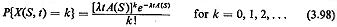Problem 3.26 applies this concept.

Example 15: Distribution of Travel Distance ("Nearest Neighbor")

Suppose that emergency response units are distributed throughout a large region as a two-dimensional Poisson process with intensity parameter y units per square mile. We wish to know the pdf of the travel distance D between an incident, whose position is selected independently of response unit positions, and the nearest response unit. Assume Euclidean travel distance. (This is sometimes known as a "nearest-neighbor" problem; in three-dimensional space this problem has been used to determine the distribution of distance between stars in a galaxy.)

Solution

We use the never-fail cumulative distribution method in conjunction with our new knowledge of spatial Poisson processes.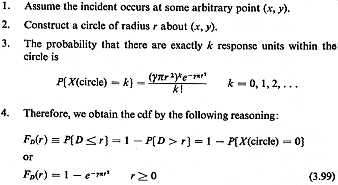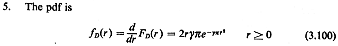This is a Rayleigh pdf with parameter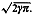Thus, the mean and variance are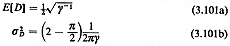Question: How could you extend these ideas to obtain other interesting properties of the system?

Example 16: Nearest Neighbor with Right-Angle Travel Distance

If travel distance is right-angle, rather than Euclidean, the analysis in Example 15 follows straight through, except instead of a circle of radius r we have a square rotated at 45°, centered at (x, y), with area equal to 2r2 . Following the same steps in the solution,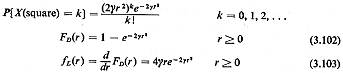This is a Rayleigh pdf with parameter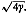The mean and variance are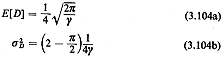Question: In Example 4 in this chapter we derived that in an isotropic environment a responseunit traveling according to the right-angle distance metric travels 4/= 1.273 times farther (on the average) than a unit traveling "as the crow flies." Thus, one might be tempted to think that the ratio of the mean right-angle to Euclidean distances computed in Examples 15 and 16 would be 1.273. In fact, the ratio is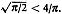. Why?

Hint: See Problems 3.9 and 3.10.

Further work: Problems 3.25 and 3.26.